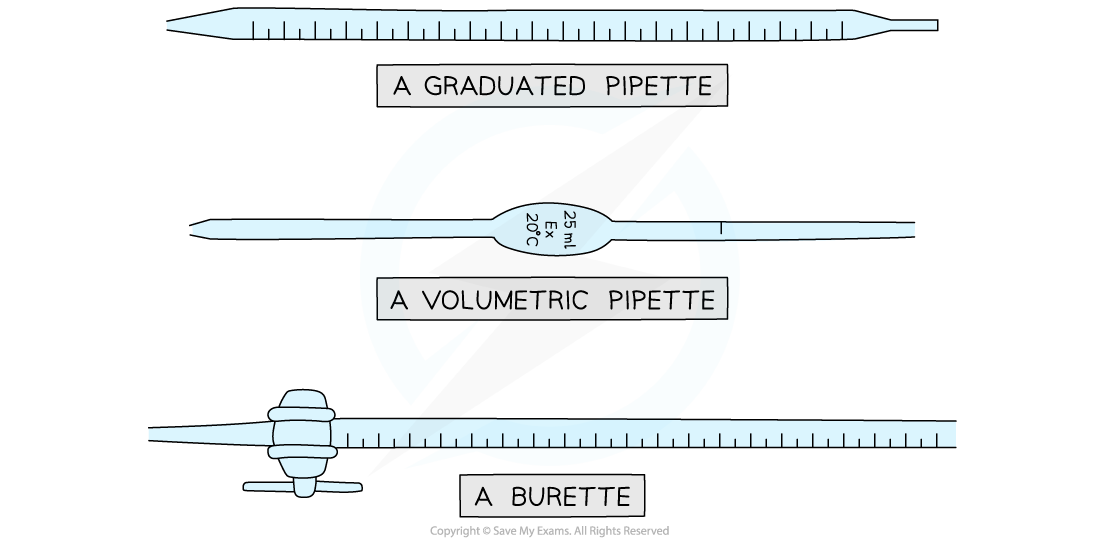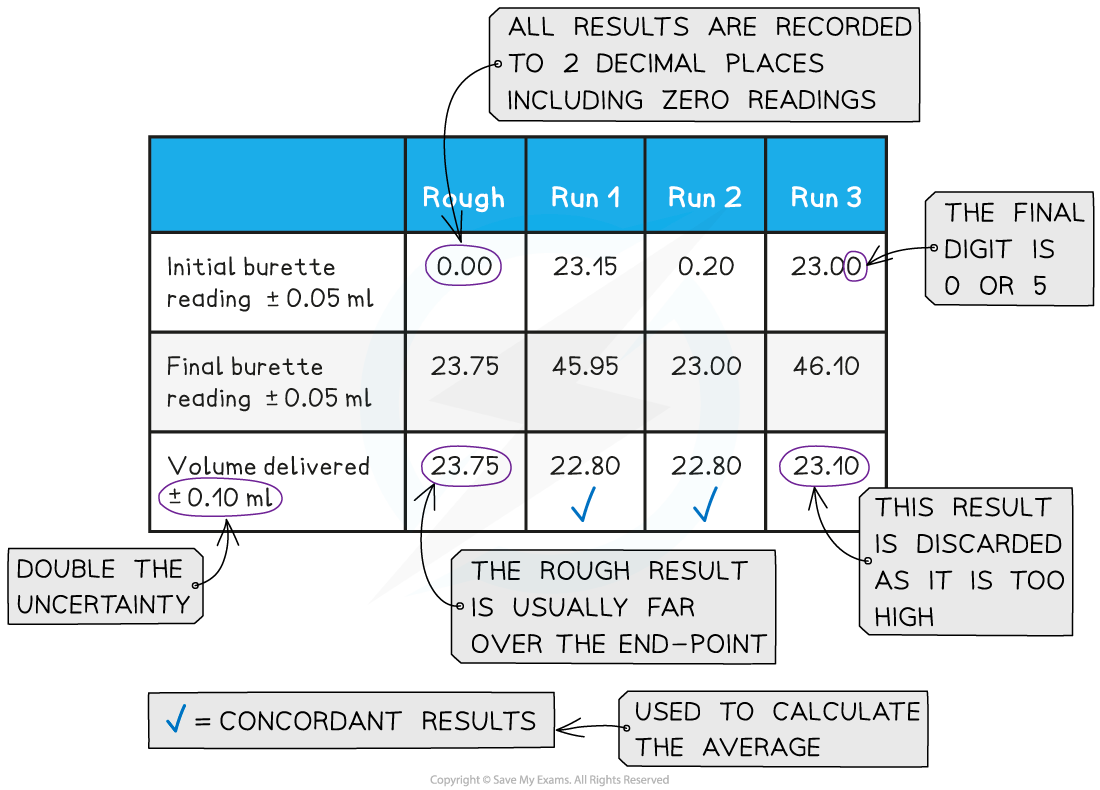# IB DP Chemistry: SL复习笔记1.2.9 Titrations

### Titrations

• Volumetric analysis is a process that uses the volume and concentration of one chemical reactant (a standard solution) to determine the concentration of another unknown solution
• The technique most commonly used is a titration
• The volumes are measured using two precise pieces of equipment, a volumetric or graduated pipette and a buretteEquipment used to measure volumes precisely in titrations

• Burettes are usually marked to a precision of 0.10 cm3
• Since they are analogue instruments, the uncertainty is recorded to half the smallest marking, in other words to ±0.05 cm3
• The end point or equivalence point occurs when the two solutions have reacted completely and is shown with the use of an indicatorThe steps in a titration

• The steps in a titration are:
• Measuring a known volume (usually 20 or 25 cm3) of one of the solutions with a volumetric or graduated pipette and placing it into a conical flask
• The other solution is placed in the burette
• A few drops of the indicator are added
• The tap on the burette is carefully opened and the solution added, portion by portion, to the conical flask until the indicator just changes colour
• Multiple trials are carried out until concordant results are obtained

#### Recording and processing titration results

• Both the initial and final burette readings should be recorded and shown to a precision of  ±0.05 cm3, the same as the uncertaintyA typical layout and set of titration results

• The volume delivered (titre) is calculated and recorded to an uncertainty of ±0.10 cm3
• The uncertainty is doubled, because two burette readings are made to obtain the titre (V final – V initial), following the rules for propagation of uncertainties (you can find more about this in Topic 11)
• Concordant results are then averaged, and non-concordant results are discarded
• Concordance is usually considered to be a consistency of ±0.05 between results, depending on the quality of the burette
• The calculation then follows the steps given in 1.2.8 Concentration calculations

#### Exam Tip

When performing titration calculations using monoprotic acids (meaning one H+) such as HCl, the number of moles of the acid and alkali will be the same. This allows you to use the relationship

C1V1 =C2V2

where C1 and V1 are the concentration and volume of the acid and C2 and V2 are the concentration and volume of the alkali. There is no need to convert the units of volume to dm3 as this is a ratio.Simply re-arrange the formula to solve for the unknown quantity.

#### Worked Example

A 0.675 g sample of a solid acid, HA, was dissolved in distilled water and made up to 100.0 cm3 in a volumetric flask. 25.0 cm3 of this solution was titrated against 0.100 mol dm-3 NaOH solution and 12.1 cmwere required for complete reaction.Determine the molar mass of the acid.

Step 1: Write the equation for the reaction:

HA (aq) + NaOH (aq) → NaA (aq) + H2O (l)

Step 2: Calculate the number of moles of the NaOHStep 3: Deduce the number of moles of the acid

Since the acid is monoprotic the number of moles of HA is also 1.21 x 10-3 mol

This is present in 25.0 cm3 of the solution

Step 4: Scale up to find the amount in the original solutionStep 5: Calculate the molar mass#### Back titration

• back titration is a common technique used to find the concentration or amount of an unknown substance indirectly
• The principle is to carry out a reaction with the unknown substance and an excess of a further reactant such as an acid or an alkali
• The excess reactant, after reaction, is then analysed by titration and the mole ratios are used to deduce the moles or concentration of the original substance being analysed

#### Worked Example

The percentage by mass of calcium carbonate, CaCO3, in a sample of marble was determined by adding excess hydrochloric acid to ensure that all the calcium carbonate had reacted. The excess acid left was then titrated with aqueous sodium hydroxide. A student added 27.20 cm3 of 0.200 mol dm-3 HCl to 0.188 g of marble. The excess acid required 23.80 cm3 of 0.100 mol dm-3 NaOH for neutralization. Calculate the percentage of calcium carbonate in the marble.

Step 1: Write the equation for the titration reaction:

HC(aq) + NaOH (aq) → NaCl (aq) + H2O (l)

Step 2: Calculate the number of moles of the NaOH

n(NaOH) = 0.02380 dm3 x 0.100 mol dm-3 = 2.380 x 10-3 mol

Step 3: Deduce the number of moles of the excess acid

Since the reacting ratio is 1:1 the number of moles of HCis also 2.380 x 10-3 mol

Step 4: Find the amount of HCl in the original solution and then the amount reacted

n(HCl)original =  0.02720 dm3 x 0.200 mol dm-3 = 5.440 x 10-3 mol

n(HCl)reacted =  5.440 x 10-3 mol – 2.380 x 10-3 mol = 3.060 x 10-3 mol

Step 5: Write the equation for the reaction with the calcium carbonate

2HC(aq) + CaCO3 (s)   CaCl2 (aq) + CO2 (g) + H2O (l)

Step 6: Deduce the number of moles of the calcium carbonate that reacted

Since the reacting ratio is 2:1 the number of moles of CaCO3 is (3.060 x 10-3 mol) ÷ 2

n(CaCO3) = 1.530 x 10-3 mol

Step 7: Calculate the mass of calcium carbonate in the sample of marble

mass = moles x molar mass = 1.530 x 10-3 mol x 100.09 g mol-1 = 0.1531g

Step 8: Calculate the percentage of calcium carbonate in the marble#### Exam Tip

Rounding off when you take averagesWhen you have an average of burette readings that comes to three decimal places, e.g.(23.20 cm3 + 23.25 cm3) ÷ 2 = 23.225 cm3You CANNOT show more than two decimal places because that would make the average more precise than the readings. To manage this situation you need to follow a simple rule. If the last digit is between a 5 and 9 then you round up; if the digit is between 0 and 4 you round down.So in this case the value recorded would be 23.23 cm3